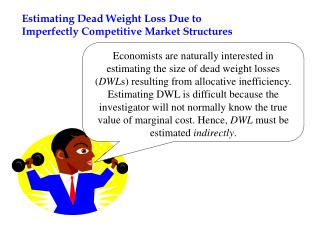# Estimating Dead Weight Loss Due to Imperfectly Competitive Market Structures - PowerPoint PPT PresentationDownload PresentationEstimating Dead Weight Loss Due to Imperfectly Competitive Market Structures

Estimating Dead Weight Loss Due to Imperfectly Competitive Market StructuresDownload Presentation## Estimating Dead Weight Loss Due to Imperfectly Competitive Market Structures

- - - - - - - - - - - - - - - - - - - - - - - - - - - E N D - - - - - - - - - - - - - - - - - - - - - - - - - - -
##### Presentation Transcript

1. Estimating Dead Weight Loss Due to Imperfectly Competitive Market Structures Economists are naturally interested in estimating the size of dead weight losses (DWLs) resulting from allocative inefficiency. Estimating DWL is difficult because the investigator will not normally know the true value of marginal cost. Hence, DWL must be estimated indirectly.

2. Harburger’s Approach1 The ABH dead weight triangle is approximated by the following equation (equation 4.1 in the text): By algebraic manipulation it can be shown that:  1Arnold Harburger. "Monopoly and Resource Allocation," American Economic Review, Dec. 1965: 77-87

3. Explanation of equation  • is price elasticity of demand d is the price cost margin, that is: P* is the monopoly price Q* is the monopoly output • To estimate d, Harburger measured the difference between rate of return for the industry and the average rate of return for all industries. • Harburger assumed that, for all industries,  = 1

4. Harburger’s estimates Based on data for U.S. industries in the 1920s, Harburger estimated the DWL due to monopoly to be equal to 1/10 of 1 percent of GNP. Hence, the welfare loss due to pricing above marginal cost is very small and would hardly justify the allocation of substantial resources for antitrust enforcement.

5. Cowling and Mueller’s Approach2 • Equation  above reveals that estimates of DWL are sensitive to assumptions made about elasticity of demand (h )Cowling and Mueller made adjustments to the methodology used by Harburger and , using a sample of 734 U.S. firms for 1963-66, reached radically different conclusions as regards the magnitude of welfare losses. • Cowling and Mueller changed a key assumption of Harburger; namely, that for all industries, h = 1. 2 Keith Cowling and Dennis Mueller. "The Social Costs of Monopoly Power," Economic Journal, December 1978: 727-48.

6. To estimate industry-level price elasticities (), Cowling and Mueller took advantage of the fact that the firm’s profit maximizing price (P*) satisfies the following condition:  Recall that d is the price-cost margin . Thus we can say:  Thus by equation , we can say: Thus if you can estimate d, you can estimate 

7. Thus substitute 1/d for  in equation  and you get:  Substituting (P*- MC)/P* for d in equation  gives us:  Thus, Cowling and Mueller showed that DWL for an industry was equal to ½ of the economic profits () of firms in the industry.

8. Figure 1 A PM Price C PC MC B D MR 0 QM QC Quantity

9. DWL is given by the black –shaded triangle. •  is given by the green-shaded rectangle P* Price MC D MR 0 Q* Quantity Measuring Dead Weight Loss

10. Back to lesson 3 Cowling and Mueller’s results • Assuming that 12 percent is a "normal" rate of return on capital, Cowling and Mueller produced 2 estimates of DWL in the U.S. economy: • The low estimate, which does not include advertising expenditures as a component of the dead weight loss, was 4 percent of GNP (about \$403 billion in 2001). • The high estimate, which reckoned advertising expenditures as "wasted resources," was 13 percent of GNP (about \$1.394 trillion in 2001). Moral of the story: If these estimates are in the ballpark, then antitrust enforcement may be an extremely cost effective way to improve social welfare